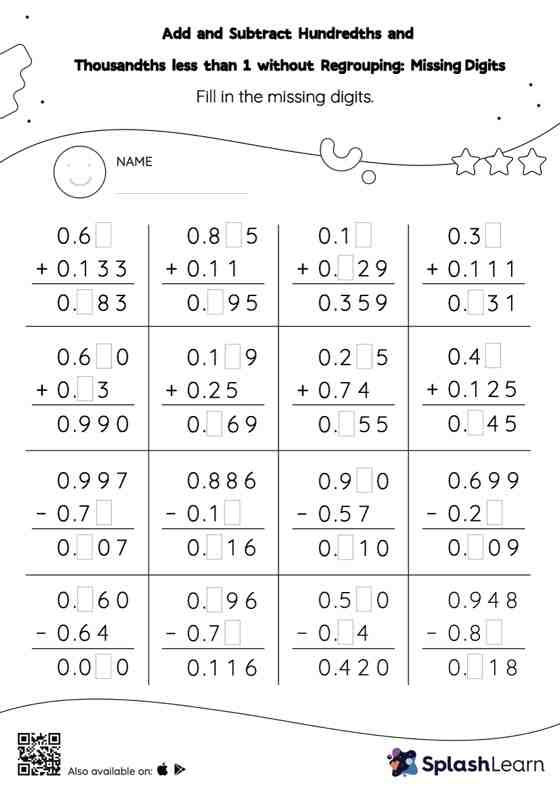# Add and Subtract Hundredths and Thousandths less than 1 without Regrouping: Missing Digits Worksheet

Home > Add and Subtract Hundredths and Thousandths less than 1 without Regrouping: Missing DigitsWhile adding and subtracting decimals, students use the relationship between addition and subtraction to find the missing number. They do not need to regroup numbers in add and subtract hundredths and thousandths less than 1 without regrouping worksheet. In each problem, the numbers are laid out in the vertical format. In this format, pair of digits in each successive place are tackled one by one. This helps students follow a structured approach.